# The time average potential of neutral hydrogen atom

## Homework Statement

[/B]
The time-averaged potential of a neutral hydrogen atom is given by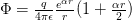where q is the magnitude of the electronic charge, and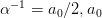being the Bohr radius. Find the distribution of charge( both continuous and discrete) that will give this potential and interpret your result physically.

## Homework Equations

[/B]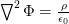## The Attempt at a Solution

[/B]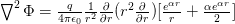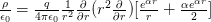since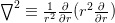andfrom product rule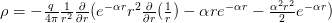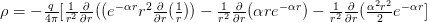now I'm stuck here no idea how to handle first term.

Last edited by a moderator:

Ok I think I figure it out.

lets take derivative one term at a time using product rule

$\frac{1}{r^{2}}\frac{\partial}{\partial r}\left[r^{2}e^{-\alpha r}\frac{\partial}{\partial r}\left(\frac{1}{r}\right)\right]=\frac{1}{r^{2}}\left[2re^{-\alpha r}\frac{\partial}{\partial r}\left(\frac{1}{r}\right)+r^{2}\left(-\alpha\right)e^{-\alpha r}\frac{\partial}{\partial r}\left(\frac{1}{r}\right)+r^{2}e^{-\alpha r}\frac{\partial^{2}}{\partial r^{2}}\left(\frac{1}{r}\right)\right]$

putting $\frac{\partial}{\partial r}\left(\frac{1}{r}\right)=-\frac{1}{r^{2}}$

$\frac{1}{r^{2}}\left[2re^{-\alpha r}\left(-\frac{1}{r^{2}}\right)+r^{2}\left(-\alpha\right)e^{-\alpha r}\left(-\frac{1}{r^{2}}\right)+r^{2}e^{-\alpha r}\frac{\partial^{2}}{\partial r^{2}}\left(\frac{1}{r}\right)\right]=\frac{1}{r^{2}}\left[-2e^{-\alpha r}\frac{1}{r}+\alpha e^{-\alpha r}+r^{2}e^{-\alpha r}\frac{\partial^{2}}{\partial r^{2}}\left(\frac{1}{r}\right)\right]$

$=-2e^{-\alpha r}\frac{1}{r^{3}}+\alpha e^{-\alpha r}\frac{1}{r^{2}}+e^{-\alpha r}\frac{\partial^{2}}{\partial r^{2}}\left(\frac{1}{r}\right)$

Last edited:
DEvens